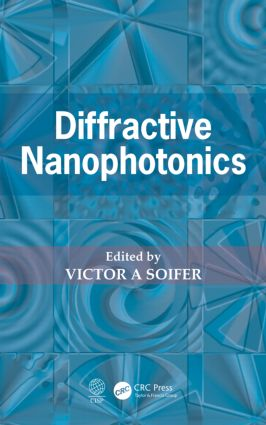Diffractive Nanophotonics

1st Edition

CRC Press

704 pages | 426 B/W Illus.

Purchasing Options:\$ = USD
Hardback: 9781466590694
pub: 2014-06-26
SAVE ~\$42.00
\$210.00
\$168.00
x
eBook (VitalSource) : 9780429169151
pub: 2016-04-19
from \$28.98

FREE Standard Shipping!

Description

Diffractive Nanophotonics demonstrates the utility of the well-established methods of diffractive computer optics in solving nanophotonics tasks. It is concerned with peculiar properties of laser light diffraction by microoptics elements with nanoscale features and light confinement in subwavelength space regions. Written by recognized experts in this field, the book covers in detail a wide variety of advanced methods for the rigorous simulation of light diffraction. The authors apply their expertise to addressing cutting-edge problems in nanophotonics.

Chapters consider the basic equations of diffractive nanophotonics and related transformations and numerical methods for solving diffraction problems under strict electromagnetic theory. They examine the diffraction of light on two-dimensional microscopic objects of arbitrary shape and present a numerical method for solving the problem of diffraction on periodic diffractive micro- and nanostructures. This method is used in modern trends in nanophotonics, such as plasmonics, metamaterials, and nanometrology. The book describes the simulation of electromagnetic waves in nanophotonic devices and discusses two methods of calculating the spatial modes of microstructured photonic crystal fibres—a relatively new class of optical fibres with the properties of photonic crystals.

The book explains the theory of paraxial and non-paraxial laser beams with axial symmetry and an orbital angular momentum—called vortex beams—which are used for optical trapping and rotating micro- and nanoparticles in a ring in the cross-sectional plane of the beam. The final chapter discusses methods for calculating the force and torque exerted by the electromagnetic field focused onto the microparticle of arbitrary form, whose dimensions are comparable with the wavelength of light.

Reviews

"The authors have offered a comprehensive and accessible reference for computational methods in difractive nanophotonics."

—Axel Mainzer in Optics & Photonics News

Basic equations of diffractive nanophotonics

Maxwell equations

Differential equations of optics

Integral theorems of optics

Integral transformations in optics

Numerical methods for diffraction theory

The finite-difference time-domain method for solving Maxwell’s equation

Numerical solution of the Helmholtz equations BPM–approach)

Diffraction on cylindrical inhomogeneities comparable to the wavelength

Analysis of diffraction on inhomogeneities by the combined finite element method and boundary element method

Finite element method for solving the two-dimensional integral diffraction equation

Diffraction of light on inhomogeneous dielectric cylinders

Fast iterative method for calculating the diffraction field of a monochromatic electromagnetic wave on a dielectric cylinder

Modelling of periodic diffractive micro- and nanostructures

The method of rigorous coupled-wave analysis for solving the diffraction problem in periodic diffractive structures

Formation of high-frequency interference patterns of surface plasma polaritons by diffraction gratings

Diffractive heterostructures with resonant magneto-optical properties

Metrology of periodic micro- and nanostructures by the reflectometry method

Photonic crystals and light focusing

One- and two-dimensional photonic crystals

Two-dimensional photonic crystal gradient Mikaelian lens

Sharp focusing of radially-polarized light

Three-dimensional photonic crystals

Interefence-litographic synthesis of photonic crystals

Three-dimensional photonic approximants of quasicrystals and related structures

One-dimensional photonic crystal based on a nanocomposite: metal nanoparticles – a dielectric

Photonic crystal fibres

Calculation of modes of photonic crystal fibres by the method of matched sinusoidal modes

Calculation of modes of photonic-crystal light guides by the finite difference method

Singular optics and superresolution

Optical elements that form wavefronts with helical phase singularities

The spiral phase plate

Quantized SPP with a restricted aperture, illuminated by a plane wave

Helical conical axicon

Helical logarithmic axicon

Elliptic vortex beams

The vortex beams in optical fibres

Matrices of optical vortices

Simulation of an optical vortex generated by a plane wave diffracted by a spiral phase plate

Optical trapping and manipulation of micro- and nano-objects

Calculation of the force acting on the micro-object by a focused laser beam

Methods for calculating the torque acting on a micro-object by a focused laser beam

A geometrical optics method for calculating the force acting by light on a microscopic object

Rotation of micro-objects in a Bessel beam

Optical rotation using a multiorder spiral phase plate

Rotation of microscopic objects in a vortex light ring formed by an axicon

Optical rotation in a double light ring

Optical rotation in a double ring of light

Rotation of micro-objects by means of hypergeometric beams and beams that do not have the orbital angular momentum using the spatial light modulator (SLM)

Investigation of rotation of micro-objects in light beams with orbital angular momentum

The capture of micro-objects in Airy beams with ballistic properties

Conclusion

Appendix A

Simulation using FULLWAVE

Appendix B

Simulation using FIMMWAVE

Appendix C

Simulation using OLYMPIOS program

Index